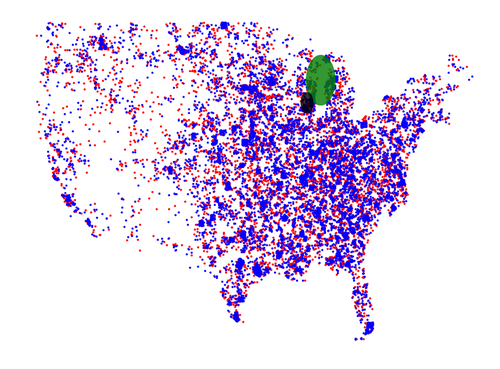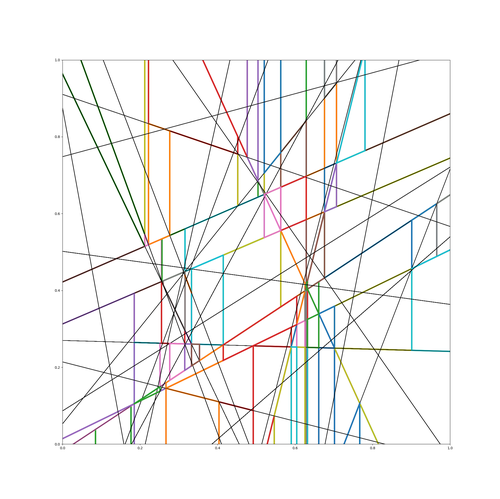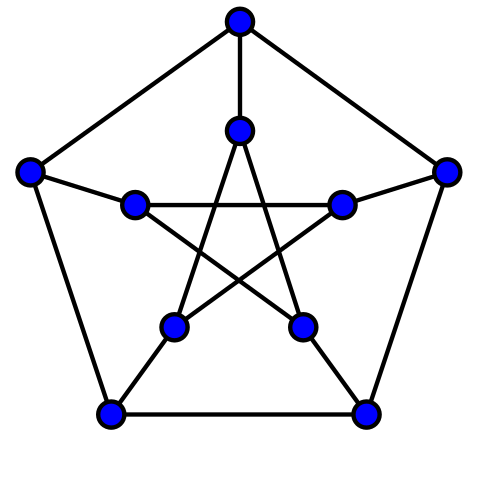# Projects

## pyscan

Implementations of a number of spatial scan statistic algorithms for different kinds of data sets.## pypartition

This is the code used for the paper Practical Low-Dimensional Halfspace Range Space Sampling.## FJLT

An implementation of the Fast Johnson Lindenstrauss Transform written in python. Uses the fast hadamard transform, numpy, and scipy libraries.

## Moore

Does a combinatorial search to find Moore Graphs that satisfy the Hoffman–Singleton theorem.## Reddit Comment Clustering

Computes clusters of subreddits using various metrics and various clustering schemes